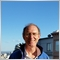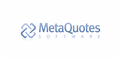# Print numbes above Price bar TD_sequential indicator220

Please advise on how to change code to cause indicator to print numbers above price bar rather than within the body. this is the MAB_TD_sequential indicator available for download.  Please be specific as i am not a programmer.  I assume after the changes are made, i recompile, and it should go into effect in the chart ?

I assume it might be in the line that starts with Object Create, but what changes would need to be made.

```//+------------------------------------------------------------------+
//|                        MAB_TD_Sequential                         |
//|                              v1.2                                |
//|                                                                  |
//|   Indicator based off of Tom DeMarks' TD Sequential indicator as |
//|   described in Jason Perl's book "DeMark Indicators"             |
//|      This is also the basis of the 5-9 indicator in Wave59.      |                                                      |
//|   05/22/2009 - Created Buy and Sell Setup along with TDST lines  |
//|               and set up criteria for arrow and alert to appear  |
//|               for a perfect setup.                               |
//|      issues:  TDST line starts updating before a full setup is   |
//|      still needs:  Countdown criteria                            |
//|                                                                  |
//|   05/26/2009 - Added the Countdown criteria.  Countdown will also|
//|               create a "+" when 13 is reach but not perfected.   |
//|      still needs:  Countdown recycle criteria(currently recycles |
//|                  automatically when setup completed)             |
//+------------------------------------------------------------------+

#property indicator_chart_window
#property indicator_buffers 6
#property indicator_color1 Red
#property indicator_color2 Green
#property indicator_color3 MediumSpringGreen
#property indicator_color4 MediumSpringGreen
#property indicator_color5 Gold
#property indicator_color6 Gold

extern color Setup=MediumSpringGreen;
extern color Countdown=Gold;

extern int NumBars=1000;
extern int Space=50;    //space above or below high to place number

//+------------------------------------------------------------------+
//| Custom indicator initialization function                         |
//+------------------------------------------------------------------+
int init()
{
//---- indicators
//TDST lines
SetIndexBuffer(0,Support);
SetIndexStyle(0,DRAW_LINE,STYLE_DOT,1);
SetIndexEmptyValue(0,EMPTY_VALUE);
SetIndexBuffer(1,Resistance);
SetIndexStyle(1,DRAW_LINE,STYLE_DOT,1);
SetIndexEmptyValue(1,EMPTY_VALUE);

//Perfected Setup
SetIndexBuffer(2,bPerfected);
SetIndexStyle(2,DRAW_ARROW);
SetIndexArrow(2,225);
SetIndexBuffer(3,sPerfected);
SetIndexStyle(3,DRAW_ARROW);
SetIndexArrow(3,226);

//Countdown
SetIndexStyle(4,DRAW_ARROW);
SetIndexArrow(4,225);
SetIndexBuffer(5,Sell);
SetIndexStyle(5,DRAW_ARROW);
SetIndexArrow(5,226);
//----
return(0);
}
//+------------------------------------------------------------------+
//| Custom indicator deinitialization function                       |
//+------------------------------------------------------------------+
int deinit()
{
//----
for(int i=0;i<Bars;i++)
{
ObjectDelete(""+i);
ObjectDelete("cd"+i);
}
//----
return(0);
}
//+------------------------------------------------------------------+
//| Custom indicator iteration function                              |
//+------------------------------------------------------------------+
int start()
{
int counted_bars=IndicatorCounted();
if(counted_bars < 0)  return(-1);
if(counted_bars>0) counted_bars--;
int limit=Bars-counted_bars;
if(counted_bars==0) limit-=1+8;
if(limit<NumBars) return(-1);

int bSetup,sSetup,bCountdown,sCountdown;
int x;
double tfm=Space*Point;
double CountTest;
bool bSetupInd,sSetupInd,bPerfect,sPerfect;
//----
for(int i=NumBars; i>=0; i--)
{
ObjectDelete(""+i);
ObjectDelete("cd"+i);
//+------------------------------------------------------------------+
//+------------------------------------------------------------------+
if(Close[i]<=Close[i+4] && Close[i+1]>=Close[i+5] && bSetup==0)//start setup
{
bSetup++;
ObjectCreate(""+i,OBJ_TEXT,0,Time[i],Low[i]-tfm);
ObjectSetText(""+i,""+bSetup,8,"Arial",Setup);
}
if(Close[i]<Close[i+4] && bSetup!=0 && ObjectFind(""+i)==-1)
{
bSetup++;
if(bSetup==9)
{
ObjectCreate(""+i,OBJ_TEXT,0,Time[i],Low[i]-tfm);
ObjectSetText(""+i,""+bSetup,10,"Arial Black",Setup);
bSetup=0;
bSetupInd=true;
sSetupInd=false;
sCountdown=0;
}
else
{
ObjectCreate(""+i,OBJ_TEXT,0,Time[i],Low[i]-tfm);
ObjectSetText(""+i,""+bSetup,8,"Arial",Setup);
}
}
//if setup is completed look for criteria for perfect setup
if(bSetupInd==true)
{
if(Low[i+1]<=Low[i+3] || Low[i]<=Low[i+2])
{
bSetupInd=false;
bPerfect=true;
bPerfected[i]=Low[i]-tfm*2;
bCountdown=1;
}
{
}
}
//Deletes numbers that were created if there is a break in sequence before 9 is reached
else if(Close[i]>=Close[i+4] && bSetup!=0)
{
for(x=i+1; x<=i+bSetup+1; x++) ObjectDelete(""+x);
bSetup=0;
}
//+------------------------------------------------------------------+
//+------------------------------------------------------------------+
if(bCountdown==13 && Close[i]<=Close[i+1] && Close[i]>CountTest)
{
ObjectCreate("cd"+i,OBJ_TEXT,0,Time[i],Low[i]-tfm*2.5);
ObjectSetText("cd"+i,"+",8,"Arial",Countdown);
}
if(bCountdown==13 && Close[i]<=Close[i+1] && Close[i]<=CountTest)
{
ObjectCreate("cd"+i,OBJ_TEXT,0,Time[i],Low[i]-tfm*2.5);
ObjectSetText("cd"+i,""+bCountdown,8,"Arial",Countdown);
bCountdown=0;
{
}
}
if(bCountdown>=1 && bCountdown<13 && Close[i]<=Close[i+2])
{
if(bCountdown==8)CountTest=Close[i];
ObjectCreate("cd"+i,OBJ_TEXT,0,Time[i],Low[i]-tfm*2.5);
ObjectSetText("cd"+i,""+bCountdown,8,"Arial",Countdown);
bCountdown++;
}
//+------------------------------------------------------------------+
//| Sell Setup                                                       |
//+------------------------------------------------------------------+
if(Close[i]>=Close[i+4] && Close[i+1]<=Close[i+5] && bSetup==0)//start setup
{
sSetup++;
ObjectCreate(""+i,OBJ_TEXT,0,Time[i],High[i]+tfm);
ObjectSetText(""+i,""+sSetup,8,"Arial",Setup);
}
if(Close[i]>=Close[i+4] && sSetup!=0 && ObjectFind(""+i)==-1)
{
sSetup++;
if(sSetup==9)
{
ObjectCreate(""+i,OBJ_TEXT,0,Time[i],High[i]+tfm);
ObjectSetText(""+i,""+sSetup,10,"Arial Black",Setup);
sSetup=0;
sSetupInd=true;
bSetupInd=false;
bCountdown=0;
}
else
{
ObjectCreate(""+i,OBJ_TEXT,0,Time[i],High[i]+tfm);
ObjectSetText(""+i,""+sSetup,8,"Arial",Setup);
}
}
//Perfected Setup
if(sSetupInd==true)
{
if(Low[i+1]>=Low[i+3] || Low[i]>=Low[i+2])
{
sSetupInd=false;
sPerfect=true;
sPerfected[i]=High[i]+tfm*2;
sCountdown=1;
}
{
Alert("TD Sequential - ",Symbol()," Sell Signal Perfected");
}
}
//Deletes numbers that were created if there is a break in sequence before 9 is reached
else if(Close[i]<=Close[i+4] && sSetup!=0)
{
for(x=i+1; x<=i+sSetup; x++) ObjectDelete(""+x);
sSetup=0;
}
//+------------------------------------------------------------------+
//| Sell Countdown Setup                                             |
//+------------------------------------------------------------------+
if(sCountdown==13 && Close[i]>=Close[i+2] && Close[i]>=CountTest)
{
ObjectCreate("cd"+i,OBJ_TEXT,0,Time[i],High[i]+tfm*2.5);
ObjectSetText("cd"+i,""+sCountdown,8,"Arial",Countdown);
sCountdown=0;
Sell[i]=High[i]+tfm*4;
{
Alert("TD Sequential - ",Symbol()," Sell Countdown Completed");
}
}
if(sCountdown==13 && Close[i]>=Close[i+2] && Close[i]<CountTest)
{
ObjectCreate("cd"+i,OBJ_TEXT,0,Time[i],High[i]+tfm*2.5);
ObjectSetText("cd"+i,"+",8,"Arial",Countdown);
}
if(sCountdown>=1 && sCountdown<13 && Close[i]>=Close[i+2])
{
if(sCountdown==8)CountTest=Close[i];
ObjectCreate("cd"+i,OBJ_TEXT,0,Time[i],High[i]+tfm*2.5);
ObjectSetText("cd"+i,""+sCountdown,8,"Arial",Countdown);
sCountdown++;
}
}
//+------------------------------------------------------------------+
//| TDST Support and Resistance lines                                |
//+------------------------------------------------------------------+
for(int j=limit-8; j>=0+8; j--)
{
//TDST Support
if(Close[j]<=Close[j+4] && Close[j+1]>=Close[j+5] && Close[j-1]<=Close[j+3] && Close[j-2]<=Close[j+2] &&
Close[j-3]<=Close[j+1] && Close[j-4]<=Close[j] && Close[j-5]<=Close[j-1] && Close[j-6]<=Close[j-2] &&
Close[j-7]<=Close[j-3] && Close[j-8]<=Close[j-4])
{
Support[j]=High[j];
Support[j+1]=EMPTY_VALUE;
}
else Support[j]=Support[j+1];
//TDST Resistance
if(Close[j]>=Close[j+4] && Close[j+1]<=Close[j+5] && Close[j-1]>=Close[j+3] && Close[j-2]>=Close[j+2] &&
Close[j-3]>=Close[j+1] && Close[j-4]>=Close[j] && Close[j-5]>=Close[j-1] && Close[j-6]>=Close[j-2] &&
Close[j-7]>=Close[j-3] && Close[j-8]>=Close[j-4])
{
Resistance[j]=Low[j];
Resistance[j+1]=EMPTY_VALUE;
}
else Resistance[j]=Resistance[j+1];
}
//----
return(0);
}
//+------------------------------------------------------------------+
```MetaQuotes Software Corp.
• www.metaquotes.net
Files:
MAB.JPG 68 kb220

Oddly enough, when i apply the indicator to a different chart, eurusd, it prints quite abit above the price bar.

i would like it to always print just a little above or below the price bar.

how to get it to be consistant ?

Files:
MAB2.JPG 102 kb20228

1. Please edit your (original) post and use the CODE button (Alt-S)! (For large amounts of code, attach it.)
General rules and best pratices of the Forum. - General - MQL5 programming forum
Messages Editor

General rules and best pratices of the Forum. - General - MQL5 programming forum

3. `ObjectCreate("cd"+i,OBJ_TEXT,0,Time[i],High[i]+tfm*2.5);`
Why are you placing it 2.5 * 50 * Point; above the high of the candle, if you don't want it that high?220

William Roeder:
1. Please edit your (original) post and use the CODE button (Alt-S)! (For large amounts of code, attach it.)
General rules and best pratices of the Forum. - General - MQL5 programming forum
Messages Editor

General rules and best pratices of the Forum. - General - MQL5 programming forum

3. Why are you placing it 2.5 * 50 * Point; above the high of the candle, if you don't want it that high?

There we go, code is posted correctly.

Not sure how much of my actual question you read.

I didnt write the indicator, just downloaded it. And would like it to print the text on the chart a bit more accurately.

Problem is some numbers print in the price bar, not above. So far it seems like the solution might be found in the ObjectCreate line ?

I dont see where you get the "50" from, in your statement above, Why are you placing it 2.5 * 50 * Point;20228

Cobra5000: I dont see where you get the "50" from, in your statement above,  Why are you placing it 2.5 * 50 * Point;
```extern int Space=50;    //space above or below high to place number
⋮
double tfm=Space*Point;
⋮
ObjectCreate("cd"+i,OBJ_TEXT,0,Time[i],High[i]+tfm*2.5);
```220

William, I appreciate that you take time to comment.

you are being rather snooty, which is the first person here like that i have encountered in this community.